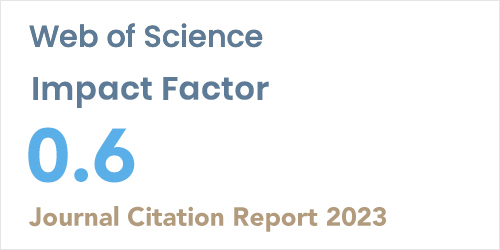ISSN: 1304-7191 | E-ISSN: 1304-7205
Volume: 41 Issue: 5 Year: 2023Higher order theory based analysis of laminated composite plates using functions trigonometric and trigonometric-hyperbolic
1Laboratory mechanics of structure and solids (LMSS),Mechanical engineering department, Faculty of Technology,Universityof SidiBel Abbes
2AlgeriaLaboratoryof Mechanical, Modeling and Experimentation,(L2ME),Facultyof Technology,University Tahri Mohammed of Bechar,Algeria
Sigma J Eng Nat Sci - DOI: 10.14744/sigma.2023.00048

#### Abstract

This work studied in detail for the first time the bending of laminated composite plates subjected t mechanical variations by new theory Trigonometric and Trigonometric-Hyperbolic functions of shear deformation. From the Euler-Lagrange hypothesis and the equations of the shear deformation theory, we will develop a present method.One of the most important problems of composite plates is the analysis of their bending behavior. The correct approach used to study their bending behavior includes two trigonometric and trigonometric-hyperbolic functions satisfying the null shear stress condition at the free edges. In this paper the bending problem is solved analytically by developing a computational code and numerically solved by Finite Element Method. In order to simplify the study of the bending behavior, an approach taking into consideration the effect of the transverse shear deformation without the shear coefficient of correction with only four unknowns has been developed while requiring five or more unknowns for other theories. Convergence analysis has been carried and the results are compared to open literature available for plate bending analysis. The approach proves to be simple and useful in analyzing the bending behavior of composite layered plates.